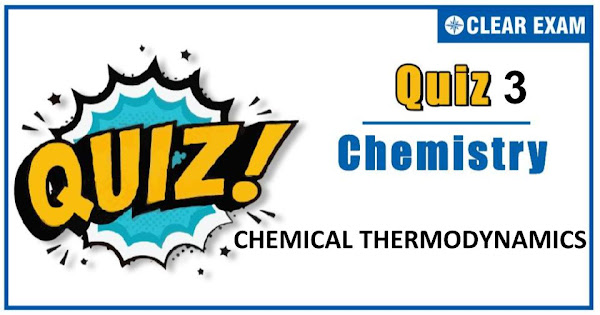## [LATEST]\$type=sticky\$show=home\$rm=0\$va=0\$count=4\$va=0

The one subject in NEET which is candidates who can easily attain good marks is Chemistry. That's the reason, often, one doesn’t pay notice and choose to compromise it. But if one wants to rank above others, the tip is to be thorough with NEET chemistry concepts. The understanding of reactions and definite basic understanding is what requires major attention in Chemistry but once done it only gets simpler from there. The main focus on the to-do list should be on getting a hang of the NCERT syllabus of NEET chemistry..

Q1. In which of the following processes of neutralization magnitude of ∆Hneutralisation is
less than that of ∆Hionisation of water?
•  HCl+NaOH
•  H2SO4+NaOH
•  CH3COOH+NaOH
•  HClO4+KOH
Solution
c) A part of heat is used in dissociation of CH3COOH, a weak acid
Q2.One mole of an ideal gas at 300K is expanded isothermally from an initial volume of 1 litre to 10 litre.
The ∆U for this process is (R=2 cal K-1mol-1):
•  163.7 cal
•  1381.1 cal
•  0.1J mol-1K-10.1Jmol-1K-1
•  1.0J mol-1K-10.1Jmol-1K-1
Solution
d) For isothermal process ∆U=0
Q3.  The bond energy of H2 is 104.3 kcal mol-1.It means:(N=Av.no.)
•   104.3 kcal heat is required to break up N bonds in N molecules of H2
•  104.3 kcal heat is required to break up N molecules to 2N atoms of H
•  104.3 kcal heat is evolved during combination of 2N atoms of H to from N molecules of H2
•  Heat of formation of H atom = 1/2 × bond energy of H—H
Solution
c) Bond energy is the amount of heat required (or evolved) to break (or form) 1 mole bond. Also,
H2 →2H ; ∆H=104.3 kcal
∴ Heat of formatin for H atom 1/2 H2→H
i.e.,equal to 1/2× 104.3 kcal

Q4. Which unit represents largest amount of energy?
•  Calorie
•  Joule
•  Erg
•  Electron volt
Solution
a) 1 cal=4.18 J=4.18 × 107 erg=4.18/1.602 × 1019eV
Q5.We believe in the laws of thermodynamics because they are
•  Theoretical
•  Derived based on mathematical analysis
•  Empirical and nobody disproved
•  Mere statements
Solution
d) Only the laws of thermodynamics are mere statements, so they, are believed.
Many mathematical expression are derived on the basis of these laws.
b) T=300 K,∆V=10-1=9 litre
∵∆H=∆U+∆PV=∆U+2×RT (∵PV=nRT)
=0+2×8.314×300=4.98 kJ
(∵∆E=0 for isothermal)
Q6. For a reversible process at T=300 K, the volume of 2 mole of ideal gas is increased from
1 litre to 10 litre, the ∆H for isothermal change is:
•  11.47 kJ
•  4.98 kJ
•  0
•  2.49 kJ
Solution
a) ∆H=HP-HR
Thus, ∆H is negative because HPR.

Q8.The Gibbs energy change and standard Gibbs energy change are equal if reaction quotient is equal to:
•  Zero
•  1
•  >1
•  <1
Solution
b) ∆G=∆G°+2.303RT log⁡Q if Q=1, ∆G=∆G°
Q9. A spontaneous change is one in which the system suffers
•  A lowering of entropy
•  No energy change
•  An increase in internal energy
•  A lowering of free energy
Solution
d) A spontaneous change is accompanied by lowering of free energy
Q10. Under the same conditions how many mL of 1M KOH and 0.5 M H2SO4 solutions, respectively
when mixed for a total volume of 100 mL produce the highest rise in temperature?
•  67:33
•  33:67
•  40:60
•  50:50
Solution
d) 50 Meq. of KOH and 50 Meq. Of H2SO4 will produce maximum heat.#### Written by: AUTHORNAME

AUTHORDESCRIPTION## Want to know more

Please fill in the details below:

## Latest NEET Articles\$type=three\$c=3\$author=hide\$comment=hide\$rm=hide\$date=hide\$snippet=hide

Name

ltr
item
BEST NEET COACHING CENTER | BEST IIT JEE COACHING INSTITUTE | BEST NEET, IIT JEE COACHING INSTITUTE: Chemical Thermodynamics Quiz-3
Chemical Thermodynamics Quiz-3
https://1.bp.blogspot.com/-IxVO_1zOjEY/X56ZiW9YxNI/AAAAAAAAIIA/Py-es1HohtMUIGU5iV8MKxaXqjwYvv6MACLcBGAsYHQ/s600/Quiz%2BImage%2B20%2B%25281%2529.jpg
https://1.bp.blogspot.com/-IxVO_1zOjEY/X56ZiW9YxNI/AAAAAAAAIIA/Py-es1HohtMUIGU5iV8MKxaXqjwYvv6MACLcBGAsYHQ/s72-c/Quiz%2BImage%2B20%2B%25281%2529.jpg
BEST NEET COACHING CENTER | BEST IIT JEE COACHING INSTITUTE | BEST NEET, IIT JEE COACHING INSTITUTE
https://www.cleariitmedical.com/2020/11/chemical-thermodynamics-quiz-3.html
https://www.cleariitmedical.com/
https://www.cleariitmedical.com/
https://www.cleariitmedical.com/2020/11/chemical-thermodynamics-quiz-3.html
true
7783647550433378923
UTF-8